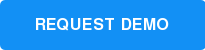3-5
JiJi Cycle

### Math Topic: Fractions

What is the relationship between fraction pies and the number line?

In this creative model, students estimate how far a set of fraction wedges will roll JiJi onto the number line.

As the level progresses, students must weigh how the impact of unit-fractions, fraction addition, and subtraction will alter the solution.

Resources: Puzzle Talk (classroom lesson)

Select level belowSee for yourself why students and educators across the country are taking a radically different approach to math.